# VIRTUAL  FLUID  LABORATORY

The objective of our project is to help students understand the experiments better without being physically requiring the experiment setup. In this Virtual Fluid Laboratory, we have added 7 experiments that we usually perform physically in any Fluid Lab.

Below is the list of experiments available to perform virtually. On opening any experiment there is a list with headings like introduction, about setup, procedure, etc. related to an experiment having explanation about the particular experiment, which can be accessed by clicking on it. We have also added 2D simulation of the experiments for generating readings and getting observations, doing calculations and producing results from that.

# Reynolds Experiment

The Reynolds experiment is among the most significant laboratory experiment in fluid mechanics that helps in distinguishing between different flow regimes. In this experiment we will identify and differentiate between Laminar, transition and turbulent flow regimes.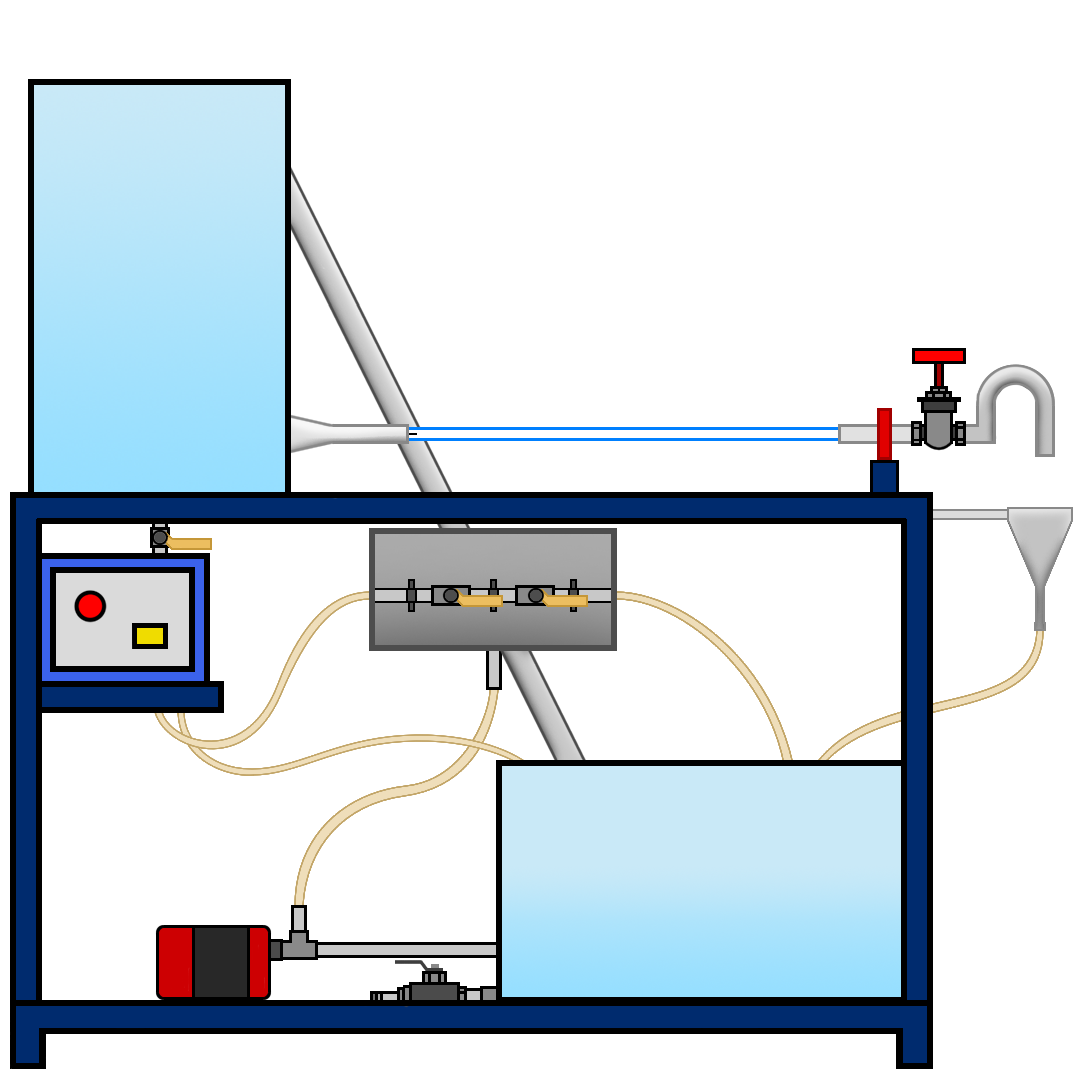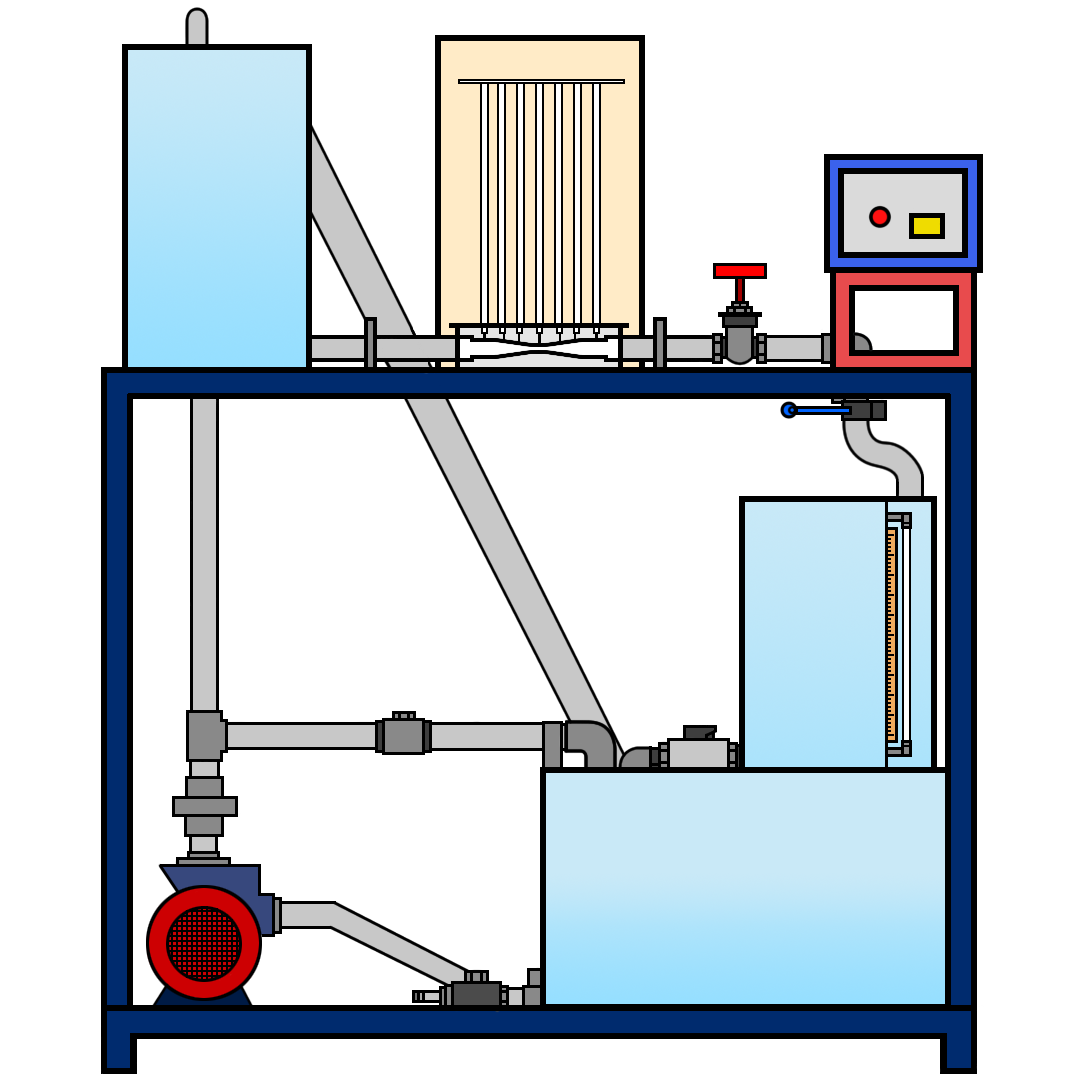# Bernoulli's Experiment

As the name suggest this is experiment to verify Bernoulli’s Theorem. In this Experiment we will calculate total energy at different points in a venturi and will plot the graph between Total Energy, Pressure Energy, Velocity Energy with respect to distance.

# Pitot Tube Apparatus

The Pitot tube is a simple and convenient instrument to measure the velocity of any point in a pipe. In this experiment we determine the velocity profile across cross section. Also, we find the coefficient of pitot tube and point velocity at centre of a tube for different flow rate.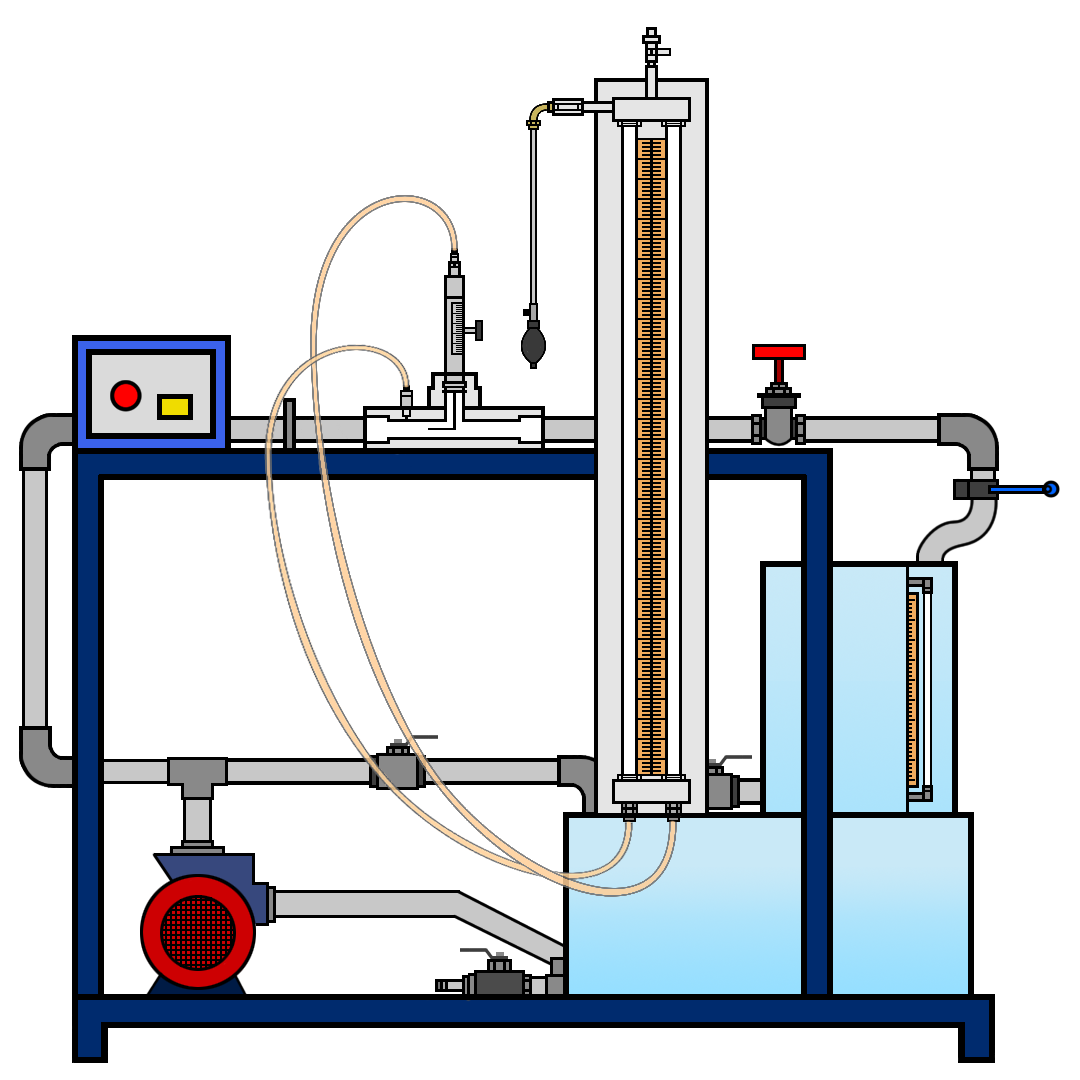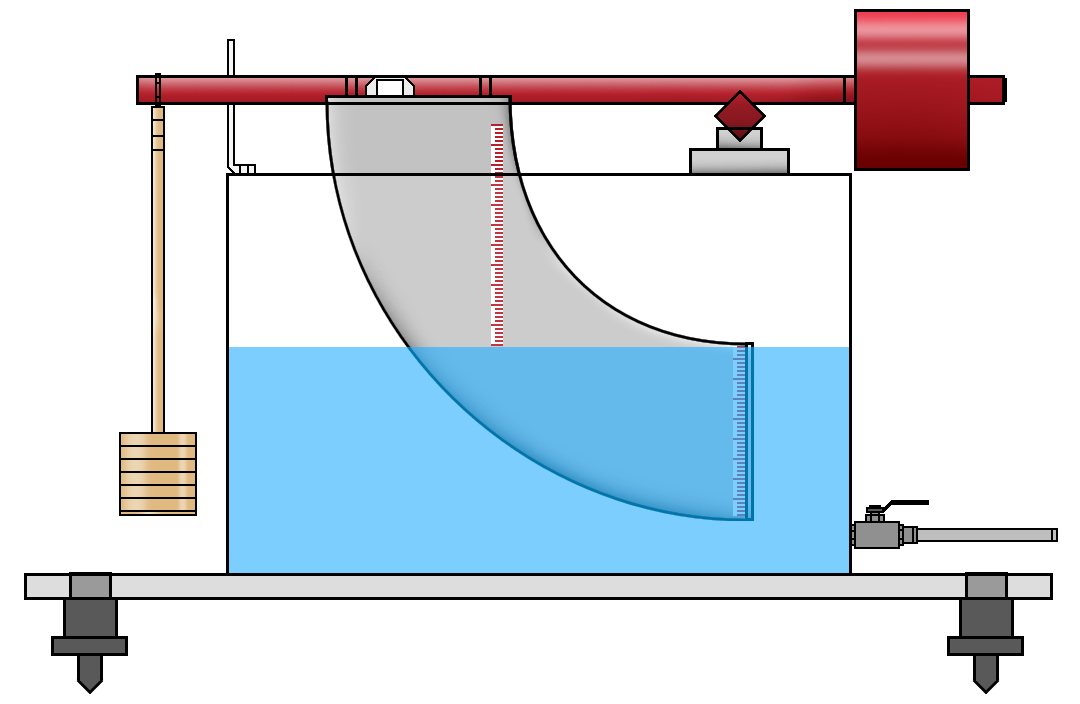# Center of Pressure

As the name suggests in this experiment, we will determine Center of Pressure and hydrostatic force on a plane surface under partial submerge and full submerge condition.

# V-Notch Experiment

A sharp crested notch is a vertical flat plate with a sharp edge which is positioned across the channel such that the fluid must flow past the sharp edge and subsequently into the pool downstream of the weir or notch plate. We will use V-Notch to estimate the discharge coefficient of a triangular notch and plot several curves in this experiment.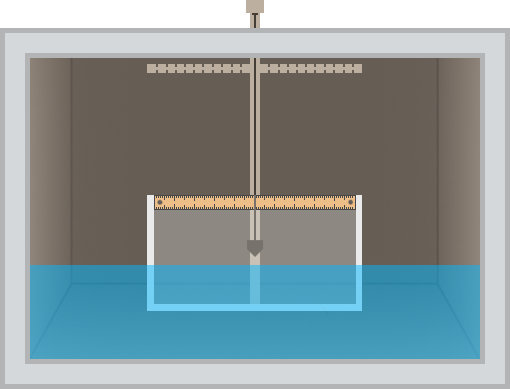# Stability of Floating Bodies

When a body is tilted at a slight angle, the point around which it is tilted tends to oscillate, and this point is known as the meta-centre. Here we are supposed to determine the meta-centric height with change in angle of heel of the given ship model.